# Electric current

An electric current is a flow of electric charge. In electric circuits this charge is often carried by moving electrons in a wire. It can also be carried by ions in an electrolyte, or by both ions and electrons such as in a plasma.

The SI unit for measuring an electric current is the ampere, which is the flow of electric charge across a surface at the rate of onecoulomb per second. Electric current is measured using a device called an ammeter.

Electric currents can have many effects, notably heating, but they also create magnetic fields, which are used in motors, inductors and generators.

## Symbol

The conventional symbol for current is I, which originates from the French phrase intensité de courant, or in English current intensity. This phrase is frequently used when discussing the value of an electric current, but modern practice often shortens this to simply current. The I symbol was used by André-Marie Ampère, after whom the unit of electric current is named, in formulating the eponymous Ampère’s force law which he discovered in 1820. The notation travelled from France to Britain, where it became standard, although at least one journal did not change from using C to I until 1896.

## ConventionsThe electrons, the charge carriers in an electrical circuit, flow in the opposite direction of the conventional electric current.The symbolfor a battery in acircuit diagram.

A flow of positive charges gives the same electric current, and has the same effect in a circuit, as an equal flow of negative charges in the opposite direction. Since current can be the flow of either positive or negative charges, or both, a convention for the direction of current which is independent of the type of charge carriers is needed. The direction of conventional current is arbitrarily defined to be the same as the direction of the flow of positive charges.

In metals, which make up the wires and other conductors in most electrical circuits, the positive charges are immobile, and the charge carriers are electrons. Because the electrons carry negative charge, their motion in a metal conductor is in the direction opposite to that of conventional current.

### Reference direction

Since the current in a wire or component can flow in either direction, when a variable I is defined to represent that current, the direction representing positive current must be specified, usually by an arrow on the circuit schematic diagram. This is called the reference direction of current I. If the current is flowing in the opposite direction, the variable I will have a negative value.

When analyzing electrical circuits, the actual direction of current through a specific circuit element is usually unknown. Consequently, the reference directions of currents are often assigned arbitrarily. When the circuit is solved, a negative value for the variable means that the actual direction of current through that circuit element is opposite that of the chosen reference direction. In electronic circuits, the reference current directions are often chosen so that all currents are toward ground. This often corresponds to the actual current direction, because in many circuits the power supply voltage is positive with respect to ground.

## Ohm’s law

Ohm’s law states that the current through a conductor between two points is directly proportional to the potential difference across the two points. Introducing the constant of proportionality, the resistance, one arrives at the usual mathematical equation that describes this relationship: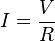$I = frac{V}{R}$

where I is the current through the conductor in units of amperes, V is the potential difference measured across the conductor in units of volts, and R is the resistance of the conductor in units of ohms. More specifically, Ohm’s law states that the R in this relation is constant, independent of the current.

## AC and DC

The abbreviations AC and DC are often used to mean simply alternating and direct, as when they modify current or voltage.

### Direct current

Direct current (DC) is the unidirectional flow of electric charge. Direct current is produced by sources such as batteries, thermocouples, solar cells, and commutator-type electric machines of the dynamo type. Direct current may flow in a conductor such as a wire, but can also flow through semiconductors, insulators, or even through a vacuum as inelectron or ion beams. The electric charge flows in a constant direction, distinguishing it from alternating current (AC). A term formerly used for direct current was galvanic current.

### Alternating current

In alternating current (AC, also ac), the movement of electric charge periodically reverses direction. In direct current (DC, also dc), the flow of electric charge is only in one direction.

AC is the form in which electric power is delivered to businesses and residences. The usual waveform of an AC power circuit is a sine wave. In certain applications, different waveforms are used, such as triangular or square waves. Audio and radio signals carried on electrical wires are also examples of alternating current. In these applications, an important goal is often the recovery of information encoded (or modulated) onto the AC signal.

## Occurrences

Natural observable examples of electrical current include lightning, static electricity, and the solar wind, the source of the polar auroras.

Man-made occurrences of electric current include the flow of conduction electrons in metal wires such as the overhead power lines that deliver electrical energy across long distances and the smaller wires within electrical and electronic equipment. Eddy currents are electric currents that occur in conductors exposed to changing magnetic fields. Similarly, electric currents occur, particularly in the surface, of conductors exposed to electromagnetic waves. When oscillating electric currents flow at the correct voltages withinradio antennas, radio waves are generated.

In electronics, other forms of electric current include the flow of electrons through resistors or through the vacuum in a vacuum tube, the flow of ions inside a battery or aneuron, and the flow of holes within a semiconductor.

## Current measurement

Current can be measured using an ammeter.

At the circuit level, there are various techniques that can be used to measure current:

• Shunt resistors
• Hall effect current sensor transducers
• Transformers (however DC cannot be measured)
• Magnetoresistive field sensors[

## Resistive heating

Main article: Joule heating

Joule heating, also known as ohmic heating and resistive heating, is the process by which the passage of an electric current through a conductor releases heat. It was first studied by James Prescott Joule in 1841. Joule immersed a length of wire in a fixed mass of water and measured the temperature rise due to a known current through the wire for a 30 minute period. By varying the current and the length of the wire he deduced that the heat produced was proportional to the square of the current multiplied by theelectrical resistance of the wire.$Q propto I^2 R$

This relationship is known as Joule’s First Law. The SI unit of energy was subsequently named the joule and given the symbol J. The commonly known unit of power, the watt, is equivalent to one joule per second.

## Electromagnetism

### ElectromagnetAccording to Ampère’s law, an electric current produces amagnetic field.

Electric current produces a magnetic field. The magnetic field can be visualized as a pattern of circular field lines surrounding the wire that persists as long as there is current.

Magnetism can also produce electric currents. When a changing magnetic field is applied to a conductor, an Electromotive force (EMF) is produced, and when there is a suitable path, this causes current.

Electric current can be directly measured with a galvanometer, but this method involves breaking the electrical circuit, which is sometimes inconvenient. Current can also be measured without breaking the circuit by detecting the magnetic field associated with the current. Devices used for this include Hall effect sensors, current clamps, current transformers, and Rogowski coils.

When an electric current flows in a suitably shaped conductor at radio frequencies radio waves can be generated. These travel at the speed of light and can cause electric currents in distant conductors.

## Conduction mechanisms in various media

In metallic solids, electric charge flows by means of electrons, from lower to higher electrical potential. In other media, any stream of charged objects (ions, for example) may constitute an electric current. To provide a definition of current that is independent of the type of charge carriers flowing, conventional current is defined to be in the same direction as positive charges. So in metals where the charge carriers (electrons) are negative, conventional current is in the opposite direction as the electrons. In conductors where the charge carriers are positive, conventional current is in the same direction as the charge carriers.

In a vacuum, a beam of ions or electrons may be formed. In other conductive materials, the electric current is due to the flow of both positively and negatively charged particles at the same time. In still others, the current is entirely due to positive charge flow. For example, the electric currents in electrolytes are flows of positively and negatively charged ions. In a common lead-acid electrochemical cell, electric currents are composed of positive hydrogen ions (protons) flowing in one direction, and negative sulfate ions flowing in the other. Electric currents in sparks or plasma are flows of electrons as well as positive and negative ions. In ice and in certain solid electrolytes, the electric current is entirely composed of flowing ions.

### Metals

A solid conductive metal contains mobile, or free electrons, which function as conduction electrons. These electrons are bound to the metal lattice but no longer to an individual atom. Metals are particularly conductive because there are a large number of these free electrons, typically one per atom in the lattice. Even with no external electric fieldapplied, these electrons move about randomly due to thermal energy but, on average, there is zero net current within the metal. At room temperature, the average speed of these random motions is 106 metres per second. Given a surface through which a metal wire passes, electrons move in both directions across the surface at an equal rate. As George Gamow wrote in his popular science book, One, Two, Three…Infinity (1947), “The metallic substances differ from all other materials by the fact that the outer shells of their atoms are bound rather loosely, and often let one of their electrons go free. Thus the interior of a metal is filled up with a large number of unattached electrons that travel aimlessly around like a crowd of displaced persons. When a metal wire is subjected to electric force applied on its opposite ends, these free electrons rush in the direction of the force, thus forming what we call an electric current.”

When a metal wire is connected across the two terminals of a DC voltage source such as a battery, the source places an electric field across the conductor. The moment contact is made, the free electrons of the conductor are forced to drift toward the positive terminal under the influence of this field. The free electrons are therefore the charge carrier in a typical solid conductor.

For a steady flow of charge through a surface, the current I (in amperes) can be calculated with the following equation:$I = {Q over t} , ,$

where Q is the electric charge transferred through the surface over a time t. If Q and t are measured in coulombs and seconds respectively, I is in amperes.

More generally, electric current can be represented as the rate at which charge flows through a given surface as:$I = frac{mathrm{d}Q}{mathrm{d}t} , .$

### Electrolytes

Electric currents in electrolytes are flows of electrically charged particles (ions). For example, if an electric field is placed across a solution of Na+ and Cl (and conditions are right) the sodium ions move towards the negative electrode (cathode), while the chloride ions move towards the positive electrode (anode). Reactions take place at both electrode surfaces, absorbing each ion.

Water-ice and certain solid electrolytes called proton conductors contain positive hydrogen ions or “protons” which are mobile. In these materials, electric currents are composed of moving protons, as opposed to the moving electrons found in metals.

In certain electrolyte mixtures, brightly coloured ions are the moving electric charges. The slow progress of the colour makes the current visible.[

### Gases and plasmas

In air and other ordinary gases below the breakdown field, the dominant source of electrical conduction is via relatively few mobile ions produced by radioactive gases, ultraviolet light, or cosmic rays. Since the electrical conductivity is low, gases are dielectrics or insulators. However, once the applied electric field approaches the breakdownvalue, free electrons become sufficiently accelerated by the electric field to create additional free electrons by colliding, and ionizing, neutral gas atoms or molecules in a process called avalanche breakdown. The breakdown process forms a plasma that contains enough mobile electrons and positive ions to make it an electrical conductor. In the process, it forms a light emitting conductive path, such as a spark, arc or lightning.

Plasma is the state of matter where some of the electrons in a gas are stripped or “ionized” from their molecules or atoms. A plasma can be formed by high temperature, or by application of a high electric or alternating magnetic field as noted above. Due to their lower mass, the electrons in a plasma accelerate more quickly in response to an electric field than the heavier positive ions, and hence carry the bulk of the current. The free ions recombine to create new chemical compounds (for example, breaking atmospheric oxygen into single oxygen [O2 → 2O], which then recombine creating ozone [O3]).

### Vacuum

Since a “perfect vacuum” contains no charged particles, it normally behaves as a perfect insulator. However, metal electrode surfaces can cause a region of the vacuum to become conductive by injecting free electrons or ions through either field electron emission or thermionic emission. Thermionic emission occurs when the thermal energy exceeds the metal’s work function, while field electron emission occurs when the electric field at the surface of the metal is high enough to cause tunneling, which results in the ejection of free electrons from the metal into the vacuum. Externally heated electrodes are often used to generate an electron cloud as in the filament or indirectly heated cathode of vacuum tubes. Cold electrodes can also spontaneously produce electron clouds via thermionic emission when small incandescent regions (called cathode spots oranode spots) are formed. These are incandescent regions of the electrode surface that are created by a localized high current. These regions may be initiated by field electron emission, but are then sustained by localized thermionic emission once a vacuum arc forms. These small electron-emitting regions can form quite rapidly, even explosively, on a metal surface subjected to a high electrical field. Vacuum tubes and sprytrons are some of the electronic switching and amplifying devices based on vacuum conductivity.

### Superconductivity

Main article: Superconductivity

Superconductivity is a phenomenon of exactly zero electrical resistance and expulsion of magnetic fields occurring in certain materials when cooled below a characteristic critical temperature. It was discovered by Heike Kamerlingh Onnes on April 8, 1911 in Leiden. Like ferromagnetism and atomic spectral lines, superconductivity is a quantum mechanical phenomenon. It is characterized by the Meissner effect, the complete ejection of magnetic field lines from the interior of the superconductor as it transitions into the superconducting state. The occurrence of the Meissner effect indicates that superconductivity cannot be understood simply as the idealization of perfect conductivity in classical physics.

### Semiconductor

In a semiconductor it is sometimes useful to think of the current as due to the flow of positive “holes” (the mobile positive charge carriers that are places where the semiconductor crystal is missing a valence electron). This is the case in a p-type semiconductor. A semiconductor has electrical conductivity intermediate in magnitude between that of a conductor and an insulator. This means a conductivity roughly in the range of 10−2 to 104 siemens per centimeter (S⋅cm−1).

In the classic crystalline semiconductors, electrons can have energies only within certain bands (i.e. ranges of levels of energy). Energetically, these bands are located between the energy of the ground state, the state in which electrons are tightly bound to the atomic nuclei of the material, and the free electron energy, the latter describing the energy required for an electron to escape entirely from the material. The energy bands each correspond to a large number of discrete quantum states of the electrons, and most of the states with low energy (closer to the nucleus) are occupied, up to a particular band called the valence band. Semiconductors and insulators are distinguished from metalsbecause the valence band in any given metal is nearly filled with electrons under usual operating conditions, while very few (semiconductor) or virtually none (insulator) of them are available in the conduction band, the band immediately above the valence band.

The ease with which electrons in the semiconductor can be excited from the valence band to the conduction band depends on the band gap between the bands. The size of this energy bandgap serves as an arbitrary dividing line (roughly 4 eV) between semiconductors and insulators.

With covalent bonds, an electron moves by hopping to a neighboring bond. The Pauli exclusion principle requires the electron to be lifted into the higher anti-bonding state of that bond. For delocalized states, for example in one dimension – that is in a nanowire, for every energy there is a state with electrons flowing in one direction and another state with the electrons flowing in the other. For a net current to flow, more states for one direction than for the other direction must be occupied. For this to occur, energy is required, as in the semiconductor the next higher states lie above the band gap. Often this is stated as: full bands do not contribute to the electrical conductivity. However, as the temperature of a semiconductor rises above absolute zero, there is more energy in the semiconductor to spend on lattice vibration and on exciting electrons into the conduction band. The current-carrying electrons in the conduction band are known as “free electrons”, although they are often simply called “electrons” if context allows this usage to be clear.

## Current density and Ohm’s law

Current density is a measure of the density of an electric current. It is defined as a vector whose magnitude is the electric current per cross-sectional area. In SI units, the current density is measured in amperes per square metre.$I=intvec Jcdot dvec A$

where$I$ is current in the conductor,$vec J$ is the current density, and$dvec A$ is the differential cross-sectional area vector.

The current density (current per unit area)$vec J$ in materials with finite resistance is directly proportional to the electric field$vec E$ in the medium. The proportionality constant is called the conductivity$sigma$ of the material, whose value depends on the material concerned and, in general, is dependent on the temperature of the material: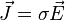$vec J = sigma vec E,$

The reciprocal of the conductivity$sigma$ of the material is called the resistivity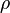$rho$ of the material and the above equation, when written in terms of resistivity becomes:$vec J = frac {vec E}{rho}$ or$vec E=rhovec J$

Conduction in semiconductor devices may occur by a combination of drift and diffusion, which is proportional to diffusion constant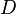$D$ and charge density$alpha_q$. The current density is then:$J =sigma E + D q nabla n,$

with$q$ being the elementary charge and$n$ the electron density. The carriers move in the direction of decreasing concentration, so for electrons a positive current results for a positive density gradient. If the carriers are holes, replace electron density$n$ by the negative of the hole density$p$.

In linear anisotropic materials, σ, ρ and D are tensors.

In linear materials such as metals, and under low frequencies, the current density across the conductor surface is uniform. In such conditions, Ohm’s law states that the current is directly proportional to the potential difference between two ends (across) of that metal (ideal) resistor (or other ohmic device):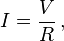$I = {V over R} , ,$

where$I$ is the current, measured in amperes;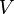$V$ is the potential difference, measured in volts; and$R$ is the resistance, measured in ohms. For alternating currents, especially at higher frequencies, skin effect causes the current to spread unevenly across the conductor cross-section, with higher density near the surface, thus increasing the apparent resistance.

## Drift speed

The mobile charged particles within a conductor move constantly in random directions, like the particles of a gas. In order for there to be a net flow of charge, the particles must also move together with an average drift rate. Electrons are the charge carriers in metals and they follow an erratic path, bouncing from atom to atom, but generally drifting in the opposite direction of the electric field. The speed at which they drift can be calculated from the equation: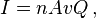$I=nAvQ , ,$

where$I$ is the electric current$n$ is number of charged particles per unit volume (or charge carrier density)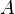$A$ is the cross-sectional area of the conductor$v$ is the drift velocity, and$Q$ is the charge on each particle.

Typically, electric charges in solids flow slowly. For example, in a copper wire of cross-section 0.5 mm2, carrying a current of 5 A, the drift velocity of the electrons is on the order of a millimetre per second. To take a different example, in the near-vacuum inside a cathode ray tube, the electrons travel in near-straight lines at about a tenth of thespeed of light.

Any accelerating electric charge, and therefore any changing electric current, gives rise to an electromagnetic wave that propagates at very high speed outside the surface of the conductor. This speed is usually a significant fraction of the speed of light, as can be deduced from Maxwell’s Equations, and is therefore many times faster than the drift velocity of the electrons. For example, in AC power lines, the waves of electromagnetic energy propagate through the space between the wires, moving from a source to a distant load, even though the electrons in the wires only move back and forth over a tiny distance.

The ratio of the speed of the electromagnetic wave to the speed of light in free space is called the velocity factor, and depends on the electromagnetic properties of the conductor and the insulating materials surrounding it, and on their shape and size.

The magnitudes (but, not the natures) of these three velocities can be illustrated by an analogy with the three similar velocities associated with gases.

• The low drift velocity of charge carriers is analogous to air motion; in other words, winds.
• The high speed of electromagnetic waves is roughly analogous to the speed of sound in a gas (these waves move through the medium much faster than any individual particles do)
• The random motion of charges is analogous to heat – the thermal velocity of randomly vibrating gas particles.

Source: WIkipedia and RCAS Library